Next: Constructing probabilistic models Up: Bayesian methods for data Previous: Bayesian methods for data   Contents

# Bayesian statistics

In the Bayesian probability theory, the probability of an event describes the observer's degree of belief on the occurrence of the event . This allows evaluating, for instance, the probability that a certain parameter in a complex model lies on a certain fixed interval.

The Bayesian way of estimating the parameters of a given model focuses around the Bayes theorem. Given some data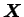and a model (or hypothesis)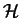for it that depends on a set of parameters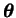, the Bayes theorem gives the posterior probability of the parameters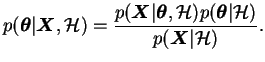(3.1)

In Equation (3.1), the term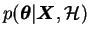is called the posterior probability of the parameters. It gives the probability of the parameters, when the data and the model are given. Therefore it contains all the information about the values of the parameters that can be extracted from the data. The term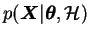is called the likelihood of the data. It is the probability of the data, when the model and its parameters are given and therefore it can usually be evaluated rather easily from the definition of the model. The term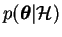is the prior probability of the parameters. It must be chosen beforehand to reflect one's prior belief of the possible values of the parameters. The last term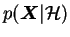is called the evidence of the model. It can be written as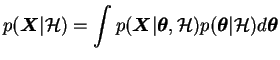(3.2)

and it ensures that the right hand side of the equation is properly scaled. In any case it is just a constant that is independent of the values of the parameters and can thus be usually ignored when inferring the values of the parameters of the model. This way the Bayes theorem can be written in a more compact form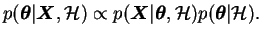(3.3)

The evidence is, however, very important when comparing different models.

The key idea in Bayesian statistics is to work with full distributions of parameters instead of single values. In calculations that require a value for a certain parameter, instead of choosing a single best'' value, one must use all the values and weight the results according to the posterior probabilities of the parameter values. This is called marginalising over the parameter.

SubsectionsNext: Constructing probabilistic models Up: Bayesian methods for data Previous: Bayesian methods for data   Contents
Antti Honkela 2001-05-30